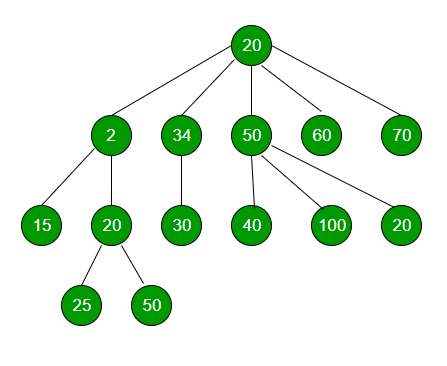# Immediate Smaller element in an N-ary Tree

Given an element x, task is to find the value of its immediate smaller element.Example :

```Input : x = 30 (for above tree)
Output : Immediate smaller element is 25
```

Explanation : Elements 2, 15, 20 and 25 are smaller than x i.e, 30, but 25 is the immediate smaller element and hence the answer.
Approach :

• Let res be the resultant node.
• Initialize the resultant Node as NULL.
• For every Node, check if data of root is greater than res, but less than x. if yes, update res.
• Recursively do the same for all nodes of the given Generic Tree.
• Return res, and res->key would be the immediate smaller element.

Below is the implementation of above approach :

 `// C++ program to find immediate Smaller ` `// Element of a given element in a n-ary tree. ` `#include ` `using` `namespace` `std; ` ` `  `// class of a node of an n-ary tree ` `class` `Node { ` ` `  `public``: ` `    ``int` `key; ` `    ``vector child; ` ` `  `    ``// constructor ` `    ``Node(``int` `data) ` `    ``{ ` `        ``key = data; ` `    ``} ` `}; ` ` `  `// Function to find immediate Smaller Element ` `// of a given number x ` `void` `immediateSmallerElementUtil(Node* root,  ` `                            ``int` `x, Node** res) ` `{ ` `    ``if` `(root == NULL) ` `        ``return``; ` ` `  `    ``// if root is greater than res, but less ` `    ``// than x, then update res ` `    ``if` `(root->key < x) ` `        ``if` `(!(*res) || (*res)->key < root->key) ` `            ``*res = root; ``// Updating res ` ` `  `    ``// Number of children of root ` `    ``int` `numChildren = root->child.size(); ` ` `  `    ``// Recursive calling for every child ` `    ``for` `(``int` `i = 0; i < numChildren; i++) ` `        ``immediateSmallerElementUtil(root->child[i], x, res); ` ` `  `    ``return``; ` `} ` ` `  `// Function to return immediate Smaller ` `// Element of x in tree ` `Node* immediateSmallerElement(Node* root, ``int` `x) ` `{ ` `    ``// resultant node ` `    ``Node* res = NULL; ` ` `  `    ``// calling helper function and using ` `    ``// pass by reference ` `    ``immediateSmallerElementUtil(root, x, &res); ` ` `  `    ``return` `res; ` `} ` ` `  `// Driver program ` `int` `main() ` `{ ` `    ``// Creating a generic tree ` `    ``Node* root = ``new` `Node(20); ` `    ``(root->child).push_back(``new` `Node(2)); ` `    ``(root->child).push_back(``new` `Node(34)); ` `    ``(root->child).push_back(``new` `Node(50)); ` `    ``(root->child).push_back(``new` `Node(60)); ` `    ``(root->child).push_back(``new` `Node(70)); ` `    ``(root->child->child).push_back(``new` `Node(15)); ` `    ``(root->child->child).push_back(``new` `Node(20)); ` `    ``(root->child->child).push_back(``new` `Node(30)); ` `    ``(root->child->child).push_back(``new` `Node(40)); ` `    ``(root->child->child).push_back(``new` `Node(100)); ` `    ``(root->child->child).push_back(``new` `Node(20)); ` `    ``(root->child->child->child).push_back(``new` `Node(25)); ` `    ``(root->child->child->child).push_back(``new` `Node(50)); ` ` `  `    ``int` `x = 30; ` ` `  `    ``cout << ``"Immediate smaller element of "` `<< x << ``" is "``; ` `    ``cout << immediateSmallerElement(root, x)->key << endl; ` ` `  `    ``return` `0; ` `} `

Output :

```Immediate smaller element of 30 is 25
```

Time Complexity : O(N), where N is the number of nodes in N-ary Tree.
Auxiliary Space : O(N), for recursive call(worst case when a node has N number of childs)

Don’t stop now and take your learning to the next level. Learn all the important concepts of Data Structures and Algorithms with the help of the most trusted course: DSA Self Paced. Become industry ready at a student-friendly price.

My Personal Notes arrow_drop_upCheck out this Author's contributed articles.

If you like GeeksforGeeks and would like to contribute, you can also write an article using contribute.geeksforgeeks.org or mail your article to contribute@geeksforgeeks.org. See your article appearing on the GeeksforGeeks main page and help other Geeks.

Please Improve this article if you find anything incorrect by clicking on the "Improve Article" button below.